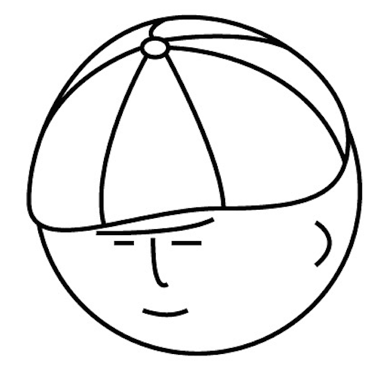マツシタのお勉強メモ

マツシタのお勉強

welcome to my engineering blog
Hacker RankFind an Element from Sorted Matrix

Problem Given an M * N matrix in which each row and each column is sorted in ascending order, write a method to find an element. Solution O(N + M) solution By traversing matrix from upper right to lower left, we can solve this problem in O…

Find the location of the given string from a sorted array of string which is interspersed with empty strings

Problem Given a sorted array of strings which is interspersed with empty strings, write a method to find the location of a given string EXAMPLE Input: find “ball” in {“at”, “”, “”, "ball”, "”, "”, "car”,“”, "”, "dad”, ”“, ”“} Output:4 Solu…

About HashTable in Java

What’s HashMap HashTable is a data structure used to implement an associative array, a structure that can map keys to values. A hash table uses a hash function to compute an index into an array. Hash table - Wikipedia Structure Of HashMap …

Print all paths which sum to a given value in a Binary Tree

Problem You are given a binary tree in which each node contains a value. Design an algorithm to print all paths which sum to a given value. The path dose not need to start or end at the root or leaf. Solution First, let’s approach this pro…